Cisco CCNA Advanced TCP/IP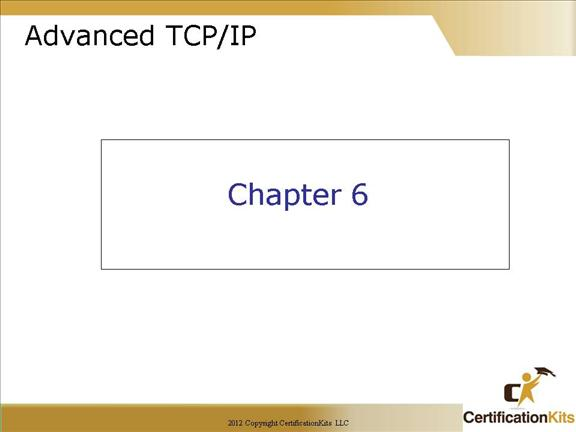This chapter will cover the following:

Class C subnetting review

Class B subnetting

VLSM design and implementation

Summarization

Cisco CCNA What Do you Know?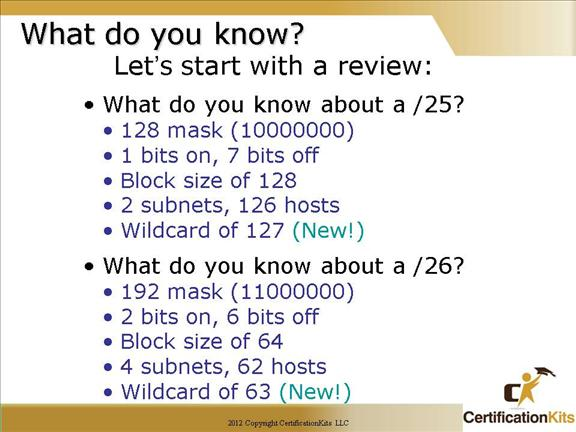These next few slides are for review purposes, plus notice we now mention the wildcard!

Cisco CCNA What do You know?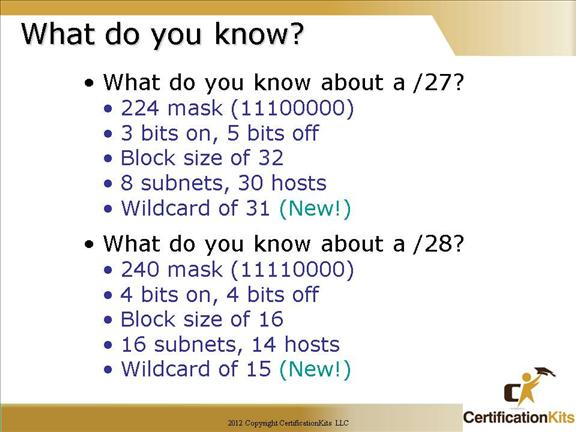Cisco CCNA What do you know?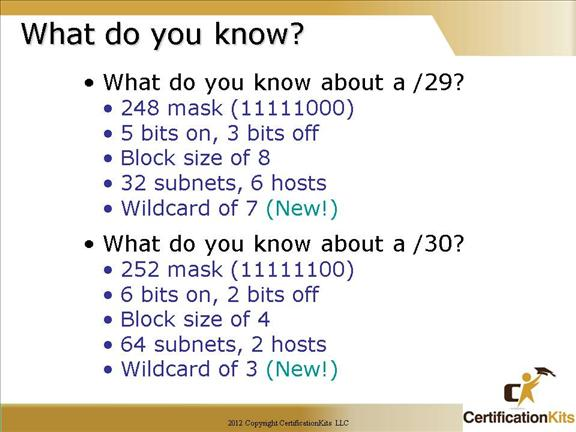Cisco CCNA Subnetting Continued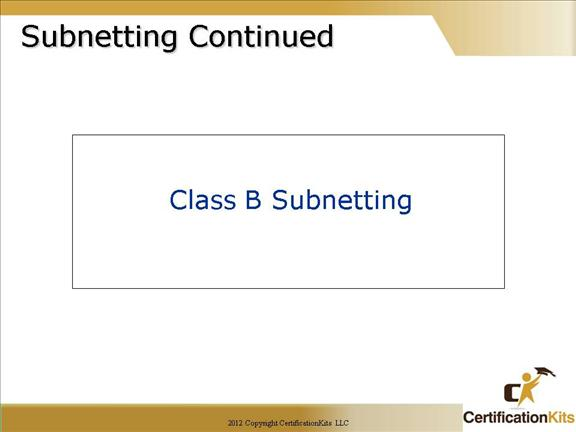We have already covered Class C subnetting.  This section will cover Class B subnetting.  The concept is identical other than the subnetting occurs starting in the third octet rather than the fourth octet as it did when subnetting Class C networks.

Cisco CCNA Class B Subnetting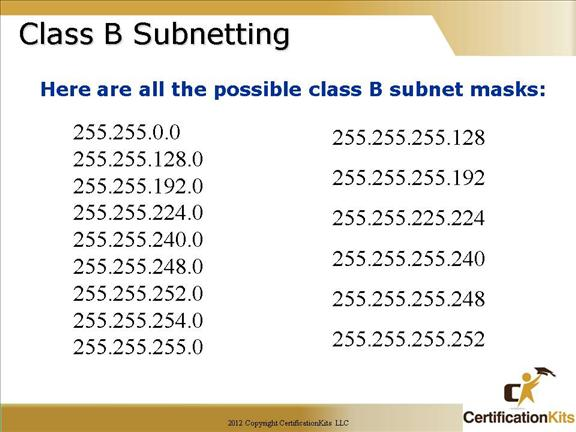Before we dive into this, let’s look at all the possible Class B subnet masks first. Notice that we have a lot more possible subnets than we do with a Class C network address.  That is because we can subnet in either the third or fourth octet with a Class B address rather than just the fourth octet with Class C addresses.

Cisco CCNA Class B Subnetting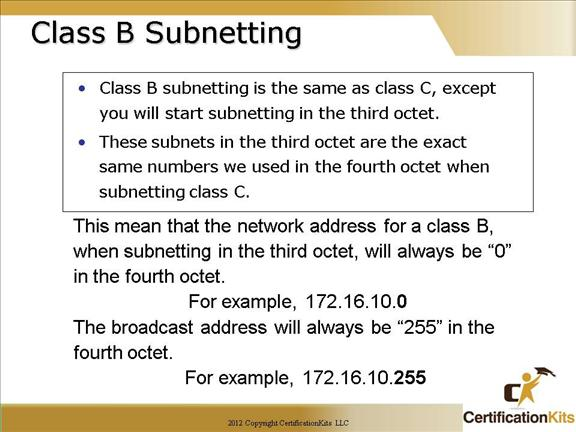Let’s look at all the possible Class B subnet masks first. Notice that we have a lot more possible subnets than we do with a Class C network address:

255.255.128.0  (/17)      255.255.255.0     (/24)

255.255.192.0  (/18)      255.255.255.128  (/25)

255.255.224.0  (/19)      255.255.255.192  (/26)

255.255.240.0  (/20)      255.255.255.224  (/27)

255.255.248.0  (/21)      255.255.255.240  (/28)

255.255.252.0  (/22)      255.255.255.248  (/29)

255.255.254.0  (/23)      255.255.255.252  (/30)

We know the Class B network address has 16 bits available for host addressing. This means we can use up to 14 bits for subnetting because we have to leave at least two bits for host addressing.

The process of subnetting a Class B network is pretty much the same as it is for a Class C, except that you just have more host bits.

Use the same subnet numbers you used with Class C, but add a zero to the network portion and a 255 to the broadcast section in the fourth octet.

Cisco CCNA Class B subnetting -/ 17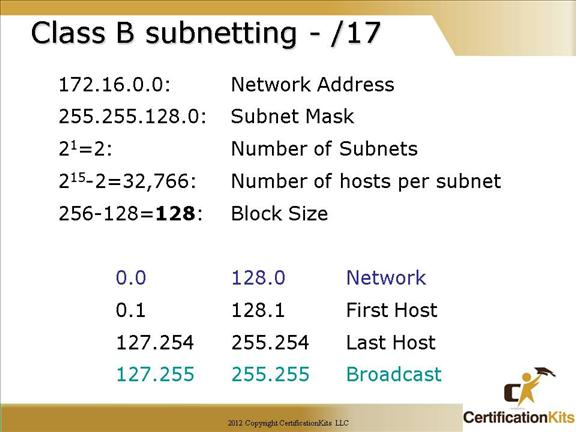172.16.0.0 = Network address

255.255.128.0 = Subnet mask

How many subnets?

Since 128 is one bit on (10000000), the answer would be 21 = 2.

How many hosts per subnet? We have 15 host bits off, 7 in the third octet and 8 in the fourth octet.  So the equation would be 215 – 2 = 32,766 hosts.

What are the valid subnets? 256 – 128 = 128, which gives us our block size.  Start with 0 and keep adding the block size to get our valid subnets.

Our valid subnets are, then, 0 and 128.

What’s the broadcast address for each subnet? The number right before the next subnet is all host bits turned on and equals the broadcast address.

What are the valid hosts? These are the numbers between the subnets and the broadcast address. The easiest way to find the hosts is to write out the subnet address and the broadcast address. This way the valid hosts are obvious.

Cisco CCNA Class B Subnetting -/20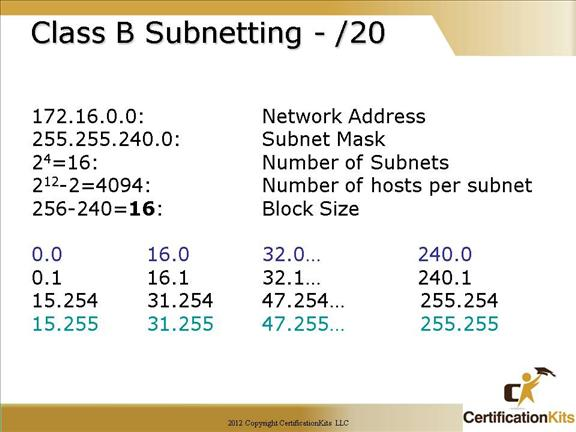172.16.0.0 = Network address

255.255.240.0 = Subnet address

Subnets? 24 = 16.

Hosts? 212 – 2 = 4094.

Valid subnets? 256 – 240 = 0, 16, 32, 48, etc., up to 224. Notice these are the same numbers as a Class C 240 mask.

Valid hosts?

172.16.0.0 = Network address

255.255.240.0 = Subnet mask

How many subnets?

Since 240 is four bits on (11110000), the answer would be 24 = 16.

How many hosts per subnet? We have 12 host bits off, 4 in the third octet and 8 in the fourth octet.  So the equation would be 212 – 2 = 4094 hosts.

What are the valid subnets? 256 – 240 = 16, which gives us our block size.  Start with 0 and keep adding the block size to get our valid subnets.

Our valid subnets are, then, 0, 16, 32, 48, 64, 80, 96, 112, 128, 144, 160, 176, 192, 208, 224 and 240.

What’s the broadcast address for each subnet? The number right before the next subnet is all host bits turned on and equals the broadcast address.

What are the valid hosts? These are the numbers between the subnets and the broadcast address. The easiest way to find the hosts is to write out the subnet address and the broadcast address. This way the valid hosts are obvious.

Cisco CCNA Class B Subnetting -/23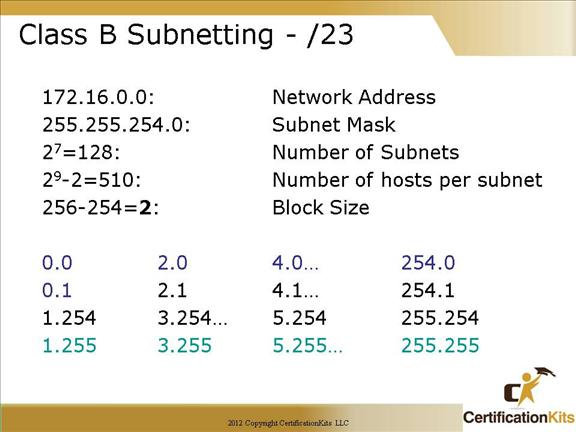172.16.0.0 = Network address

255.255.254.0 = Subnet mask

How many subnets?

Since 254 is seven bits on (11111110), the answer would be 27 = 128.

How many hosts per subnet? We have 9 host bits off, 1 in the third octet and 8 in the fourth octet.  So the equation would be 29 – 2 = 510 hosts.

What are the valid subnets? 256 – 254 = 2, which gives us our block size.  Start with 0 and keep adding the block size to get our valid subnets.

Our valid subnets are, then, 0, 2, 4, 6, 8, 10 etc. up to 254.

What’s the broadcast address for each subnet? The number right before the next subnet is all host bits turned on and equals the broadcast address.

What are the valid hosts? These are the numbers between the subnets and the broadcast address. The easiest way to find the hosts is to write out the subnet address and the broadcast address. This way the valid hosts are obvious.

Cisco CCNA Class B Subnetting -/25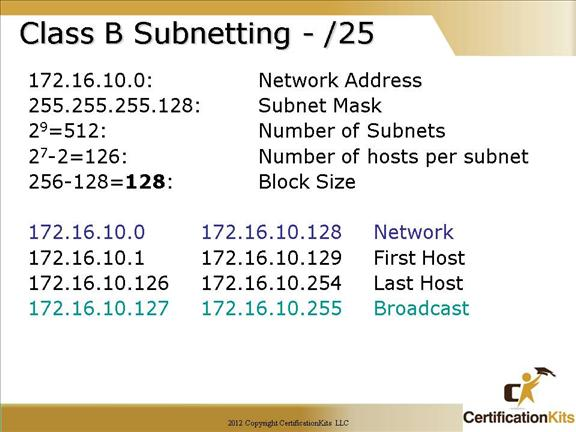172.16.0.0 = Network address

255.255.255.128 = Subnet mask

How many subnets?

Since 128 is 9 bits on, 8 in the third octet and one in the fourth octet, the answer would be 29 = 512.

How many hosts per subnet? We have 7 host bits off, all in the fourth octet.  So the equation would be 27 – 2 = 126 hosts.

What are the valid subnets?

What’s the broadcast address for each subnet? The number right before the next subnet is all host bits turned on and equals the broadcast address.

What are the valid hosts? These are the numbers between the subnets and the broadcast address. The easiest way to find the hosts is to write out the subnet address and the broadcast address. This way the valid hosts are obvious.